Next: Projective Mappings Up: Projective Space Previous: Projective Space

## Canonical Injection of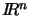into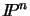Affine spacecan be embedded isomorphically inby the standard injection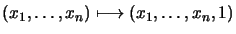. Affine points can be recovered from projective ones with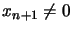by the mapping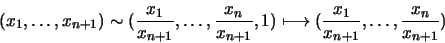A projective point with xn+1=0 corresponds to an ideal point at infinity'' in the (x1, ..., xn) direction in affine space. The set of all such infinite'' points satisfying the homogeneous linear constraint xn+1=0 behaves like a hyperplane, called the hyperplane at infinity.

However, these mappings and definitions are affine rather than projective concepts. They are only meaningful if we are told in advance that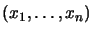represents normal'' affine space and xn+1 is a special homogenizing coordinate. In a general projective space any coordinate (or linear combination) can act as the homogenizing coordinate and all hyperplanes are equivalent -- none is especially singled out as the hyperplane at infinity''. These issues will be discussed more fully in chapter 4.

Bill Triggs
1998-11-13